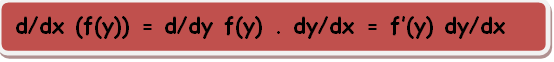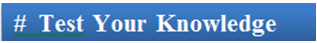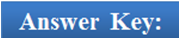×#### Thank you for registering.

One of our academic counsellors will contact you within 1 working day.

Click to Chat

1800-1023-196

+91-120-4616500

CART 0

• 0

MY CART (5)

Use Coupon: CART20 and get 20% off on all online Study Material

ITEM
DETAILS
MRP
DISCOUNT
FINAL PRICE
Total Price: Rs.

There are no items in this cart.
Continue Shopping```Differentiation of Composite, Logarithmic and Implicit Functions

Table of Content

Differentiation of Composite Functions

Differentiation of Logarithmic Functions

What is an implicit function?

Differentiation of Implicit Functions

A Direct Formula for computing the derivatives of Implicit Functions

Related ResourcesIn this section, we shall discuss the concepts of composite, logarithmic and implicit functions. First of all we shall study the nature of these functions and then we shall switch to the differentiation of these functions.

Differentiation of Composite FunctionsIf g and h are two functions defined by y = g(u) and u = h(x) respectively then a function defined by y = g [h(x)] or goh(x) is called a composite function or a function of a function.

Hence, if g(x) and h(x) are two differentiable functions, then fog is also differentiable and hence (fog)’(x) = f’(g(x)).g’(x)

This can be better explained as given below:

Let y be a differential function of u and u is a differential function of x, then

dy/dx = dy/(du) × du/(dx)

Let y = g(u) and u = f(x)

Let Δx be increment in x and Δ u and Δy be the Corresponding increments in u and y respectively.

y + Δ y = g(u + Δu) and u + Δu = f(x + Δx)

Δy = g(u + Δu) - g(u) and Δu = f(x + Δx) - f(x)

Δy/(Δu) = (g (u + Δu)/(Δu) and Δu/(Δx) = (f(x + ?x) – f(x))/?x

Δy/(Δx) = Δy/(Δu).Δu/(Δx)

Applying limits

lim?x→0 ?y/?x = lim?u→0  ?y/?u × lim?u→0  ?u/?x

⇒ dy/dx = dy/du × du/dx

= d/du g(u) × d/dx f(x)Note:

If y = t(u) and u = h(x) then dy/dx = dy/du . du/dx

In fact, this can be extended to any number of chains. Hence, if we have y = (A)B, where A is a function of x and B is a constant then we have

dy/dx = B(A)B-1 . d/dx (A).

Illustration:

Find the derivative of composite function y = sin (cot x).

Solution:

Assume cot x = u so that we have y = sin u, u = cot x

⇒ dy/dx = dy/du × du/dx

= d/du (sin u ) × d/dx (cot x)

= cos u.(- cosec2x)

= – cos (cot x). cosec2x

Differentiation of Logarithmic Functions

In order to find the derivative of:

(a) a function which is the product or quotient of a number of functions like

y = g1(x)g2(x)g3(x)........  or y = (g1(x)g2(x)g3(x)...... )/ (s1(x)s2(x)s3(x).... )

(b) a function of the form [g(x)]s(x) where g and s are both derivable, students are advised to take the logarithm of the function first followed by its differentiation.

This can also be done as y = [g(x)]s(x) = es(x). ln (g(x)) and then differentiate.

Illustration:

Find dy/dx for y = xx.

Solution:

The given function is y = xx

Taking logarithm of both the sides we have

ln y = x ln x

Now, differentiating both sides we have

d/dx (ln y) = d/dx (x ln x)

⇒ 1/y . dy/dx = x.(1/x) + ln x

= 1 + ln x

Hence, dy/dx = xx (1 + ln x)

What is an implicit function?

If two variables say u and v are associated by a relation of the form g(u, v) = 0 and it is not possible to express one of them in terms of the other i.e. we cannot express u as a function of v in the form v = ∅ (u) then the functions are said to be implicit functions.

Example: Folium is an example of implicit function. Its equation isDifferentiation of Implicit Functions

Working rule to find dy/dx i.e. implicit derivative are as follows.

Differentiate the given relation between x and y w.r.t. x

Bring all the terms containing dy/dx on left-hand side and remaining terms in right hand side and then find d/dx.

Corresponding to each and every curve that is presented by an implicit equation, there may exist one or more than one explicit functions representing the equation. As a matter of fact, dy/dx at any point on the curve remains the same whether the differentiation is done explicitly or implicitly.

Note:

?Illustration:

Differentiate the function xy = x3 + y3.

Solution:

Differentiating w.r.t. x we get

dy/dx (xy) = d/dx x3 + d/dx y3

x dy/dx y.1 = 3x2 + 3y2 d/dx

or (x – 3y2) dy/dx = 3x2 - y

dy/dx = (3x2 - y)/(x – 3y2)

A Direct Formula for computing the derivatives of Implicit Functions

Let us consider a function f(x,y) = 0. By taking all the terms of left side and putting it equal to f(x, y) we can directly compute the derivative using the following result:Illustration:

If x2 + 2xy + y3 = 4, then find dy/dx.

Solution:

The given function is x2 + 2xy + y3 = 4

Differentiating both sides w.r.t. x,

2x + 2(x dy/dx + y.1) + 3y2 dy/dx = 0

Hence, dy/dx = – 2(x + y)/(2x + 3y2)

Second Method:

The above questions can also be solved using the formula discussed above.

dy/dx = – (diff. of f w.r.t.x keeping y as constant)/(diff. of f w.r.t.y keeping x as constant)

= – (2x + 2y)/(2x + 3y2).Note:

(d2y)/(dx2) can also be written as y" or y2

It is known as second ordered differential co-efficient.

Illustration:

Evaluate limk→0, h→0 (f (a +"k +" h) – f(a + k) – f(a + h) + f(a))/(h k), where f(x) is a twice differential function.

Solution:

limk→0 1/k [limk→0 ((f (a +"k +" h) – f(a + k))/h – (f(a + h) + f(a))/k)]

= lim(k→0) 1/k [f' (a + k - f'(a))]

= f" (a)dy/dx = (dy/dt) / (dx/dt)

dy/dx = 1/(dx/dy)

d2y/dx2 ≠ (d2y/dt2) / (d2x/dt2)

d2y/dx2 ≠ 1/ (d2x/dy2)

Illustration:

If y = (sin-1 x)2, then show that (1 – x2) (d2y)/(dx2) – x dy/dx – 2 = 0.

Solution:

The given function is y = (sin-1 x)2

dy/dx = d/dx (sin-1 x)2

= 2 (sin-1x)2 -1 d/dx (sin-1x)

= (2 sin-1x)/√(1-x2 )              (-1 < x < 1)

√(1 – x(-2)) dy/dx = 2sin-1x

Differentiating again, with respect to x, we getQ1. While trying to compute the second derivative

(a) d2y/dx2 = (d2y/dt2) / (d2x/dt2)

(b) d2y/dx2 = (dy/dt) / (d2x/dt2)

(c) d2y/dx2 = d/dx (dy/dx)

(d) d2y/dx2 = (d2y/dt2) .(d2t/dx2)

Q2. The composite function (fog)’(x) is

(a) f’(g(x)).g’(x)

(b) f’(g(x)).g’(x)

(c) f’(g(x)).g’(x)

(d) f’(g(x)).g’(x)

Q3. The second derivative can also be written as

(a) d2y/ dx2 or y” or y2.

(b) d2y/ dx2 or y” or y2.

(c) dy/ dx2 or y” or y2.

(d) d2y/ dx2 or y’ or y2.

Q4. dy/dx at any point on the curve

(a) decreases when the differentiation is done explicitly and increases when it is done implicitly.

(b) increases when the differentiation is done explicitly and decreases when it is done implicitly.

(c) changes when the differentiation is done explicitly or implicitly.

(d) remains the same whether the differentiation is done explicitly or implicitly.

Q5. d/dx (f(y)) =

(a) f”(y) dy/dx

(b) f’(y) dy/dx

(c) f(y). f’(y)

(d) f’(y) d2y/dx2Q1.

Q2.

Q3.

Q4.

Q5.

(c)

(c)

(a)

(d)

(b)

Related Resources

Check here for the Complete Syllabus of IIT JEE Mathematics.

Look into the Past Year Papers with Solutions to get a hint of the kind of questions asked in the exam.

Refer theUseful Books of Mathematics here.

To read more, Buy study materials of Methods of Differentiation comprising study notes, revision notes, video lectures, previous year solved questions etc. Also browse for more study materials on Mathematics here.

```### Course Features

• 731 Video Lectures
• Revision Notes
• Previous Year Papers
• Mind Map
• Study Planner
• NCERT Solutions
• Discussion Forum
• Test paper with Video Solution